# 17. Exercises. (ii)

2.1) Consider the initial value problem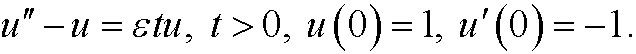Find a two-term perturbation approximation u=u(t) for2.2)
Verify the following order relations
:

a)b)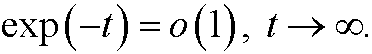c)d)2.3) Use Poincaré-Lindstedt’s method to get a two-term perturbation approximation y=y(t) to the problem2.4) Consider the initial value problem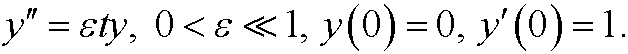Use regular perturbation methods to achieve a three-term approximate solution y=y(t) for t>0.

2.5) Show that regular perturbation fails for the boundary value problemFind the exact solution y=y(t). If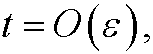show that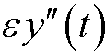is large. Ifshow that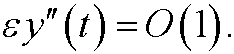Find the inner and outer approxiamtions from the exact solution.

2.6) Suppose that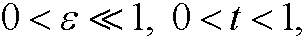and use singular perturbation to find an approximate solution y=y(t) to the problem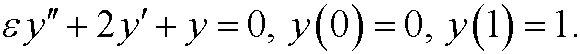2.7) Show why singular perturbation fails for the boundary value problemby comparing with the exact solution y=y(t).

2.8)
Try singular perturbation on the boundary value problemDiscuss the result.